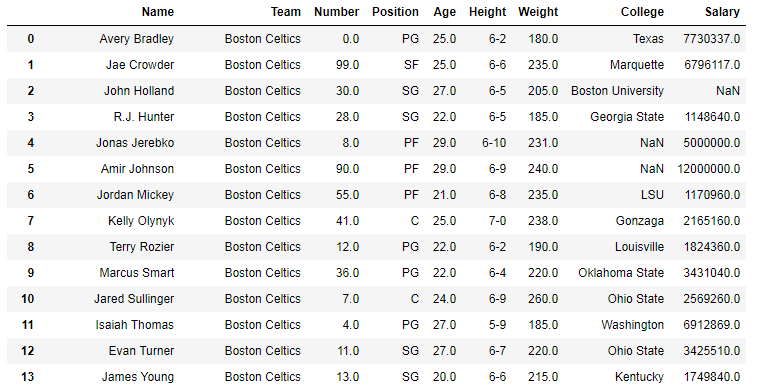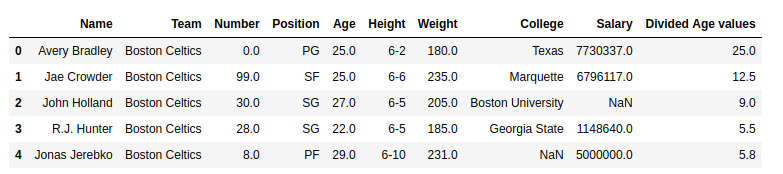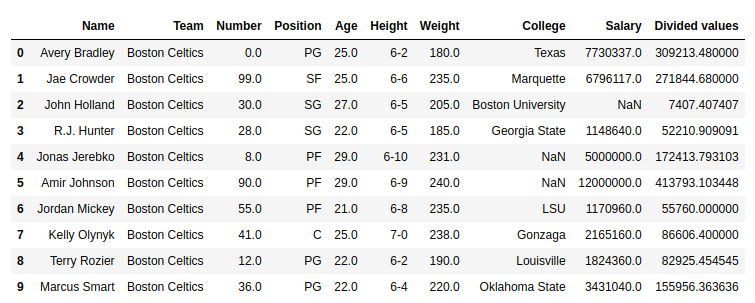Related Articles

# Python | Pandas Series.div()

• Last Updated : 01 Oct, 2018

Python is a great language for doing data analysis, primarily because of the fantastic ecosystem of data-centric Python packages. Pandas is one of those packages and makes importing and analyzing data much easier.

Python `Series.div()` is used to divide series or list like objects with same length by the caller series.

Syntax: Series.div(other, level=None, fill_value=None, axis=0)

Parameters:
other: other series or list type to be divided by the caller series
fill_value: Value to be replaced by NaN in series/list before division
level: integer value of level in case of multi index

Return type: Caller series with divided values

In the following examples, the data frame used contains data of some NBA players. The image of data frame before any operations is attached below.Example #1: Dividing Series by list

In this example, the top 5 rows are stored in new variable using .head() method. After that a list of same length is created and the age column is divided by the list column using .div() method

 `# importing pandas module ``import` `pandas as pd`` ` `# reading csv file from url ``data ``=` `pd.read_csv(``"https://media.geeksforgeeks.org/wp-content/uploads/nba.csv"``)`` ` `# creating short data of 5 rows``short_data ``=` `data.head()`` ` `# creating list with 5 values``list` `=``[``1``, ``2``, ``3``, ``4``, ``5``]`` ` `# Dividing by list data``# creating new column``short_data[``"Divided Age values"``]``=` `short_data[``"Age"``].div(``list``)`` ` `# display``short_data`

Output:
As shown in the output image, it can be compared that the Divided age value column is having the Divided values of (Age)/(list).Example #2: Dividing series by series having null values

In this example, the Salary column is divided by the Age column. Since the salary column contains null values too, by default it returns NaN no matter what is divided. In this example, 200000 is passed to replace null values with 200000.

 `# importing pandas module ``import` `pandas as pd`` ` `# reading csv file from url ``data ``=` `pd.read_csv(``"https://media.geeksforgeeks.org/wp-content/uploads/nba.csv"``)`` ` `# passing age series to variable``age ``=` `data[``"Age"``]`` ` `# na replacement``na ``=` `200000`` ` `# Dividing values``# storing to new column``data[``"Divided values"``]``=` `data[``"Salary"``].div(other ``=` `age, fill_value ``=` `na)`` ` `# display``data.head(``10``)`

Output:
As shown in the output image, the Divided values column has divided age column with 200000 in case of Null values.Attention geek! Strengthen your foundations with the Python Programming Foundation Course and learn the basics.

To begin with, your interview preparations Enhance your Data Structures concepts with the Python DS Course. And to begin with your Machine Learning Journey, join the Machine Learning – Basic Level Course

My Personal Notes arrow_drop_up